# BASIC ENGINEERING SCIENCE BASIC ENGINEERING SCIENCE ELECTROSTATIC Capacitance

• Slides: 18BASIC ENGINEERING SCIENCEBASIC ENGINEERING SCIENCE ELECTROSTATIC üCapacitance and unit üParallel-Plate capacitor üCapacitor in parallel and series üCharging and discharging of capacitors üDielectric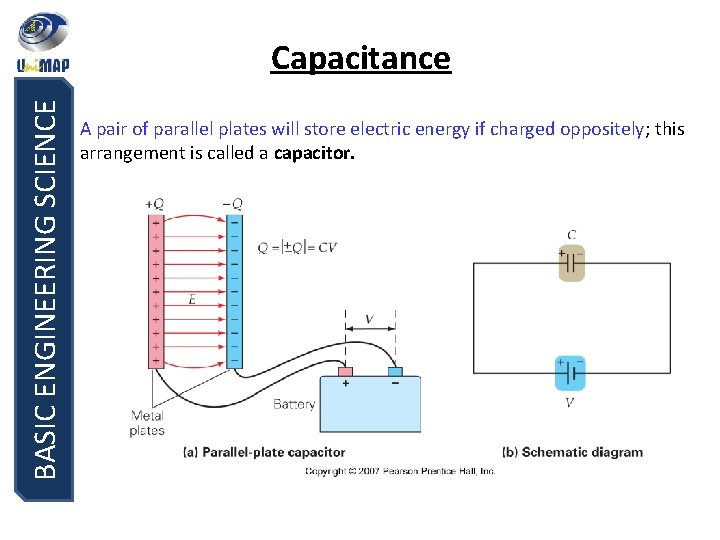BASIC ENGINEERING SCIENCE Capacitance A pair of parallel plates will store electric energy if charged oppositely; this arrangement is called a capacitor.BASIC ENGINEERING SCIENCE Capacitance The charge is related to the potential difference; the ratio is called the capacitance. SI unit of capacitance: the farad, F. For a parallel-plate capacitor, or Michael Faraday (1791 – 1867) British physicist The quantity inside the parentheses is called the permittivity of free space, ε 0.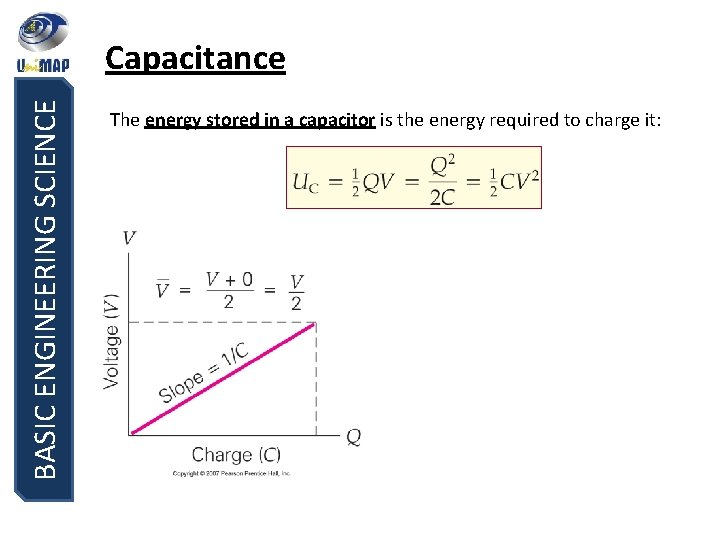BASIC ENGINEERING SCIENCE Capacitance The energy stored in a capacitor is the energy required to charge it: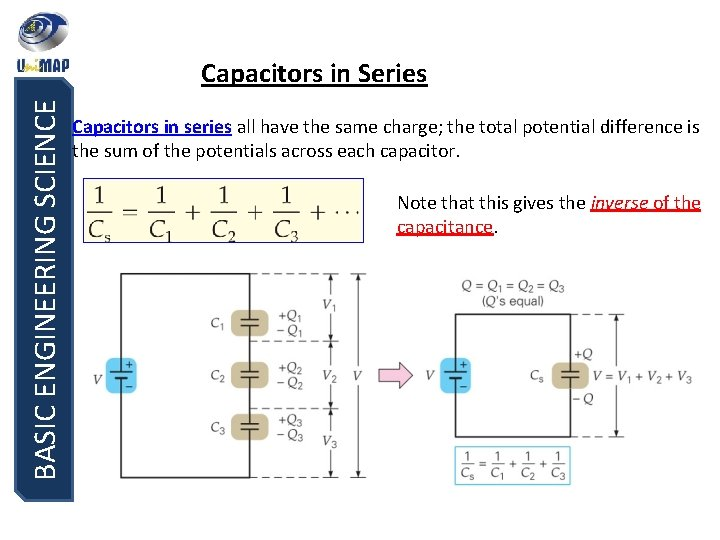BASIC ENGINEERING SCIENCE Capacitors in Series Capacitors in series all have the same charge; the total potential difference is the sum of the potentials across each capacitor. Note that this gives the inverse of the capacitance.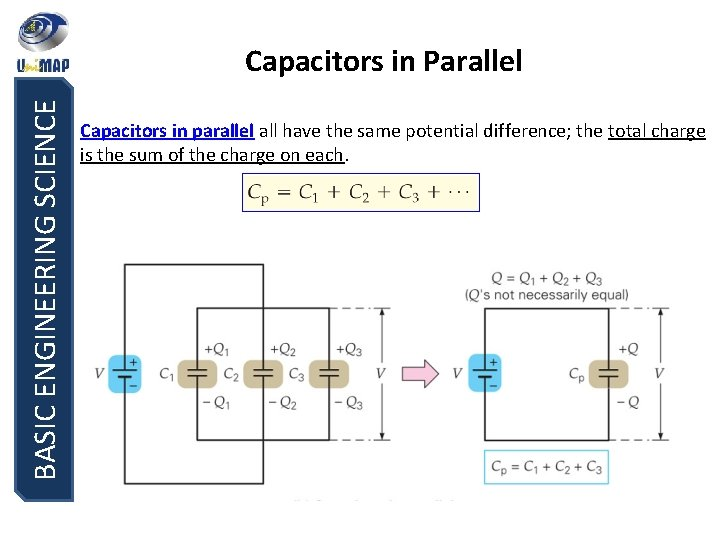BASIC ENGINEERING SCIENCE Capacitors in Parallel Capacitors in parallel all have the same potential difference; the total charge is the sum of the charge on each.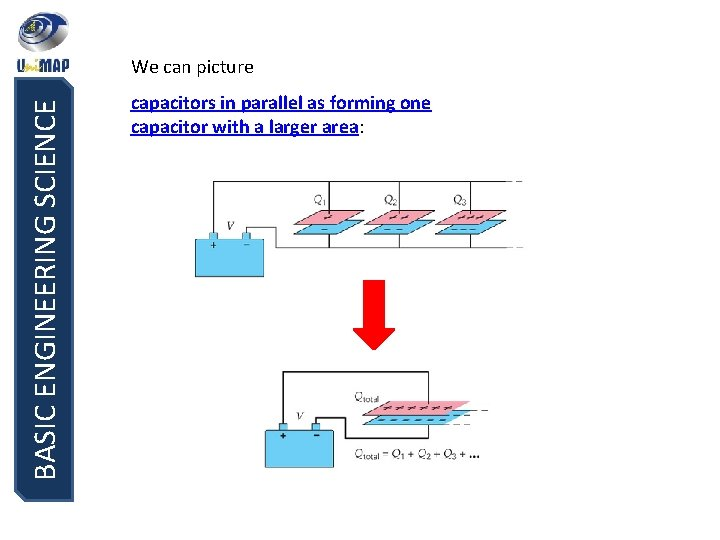BASIC ENGINEERING SCIENCE We can picture capacitors in parallel as forming one capacitor with a larger area: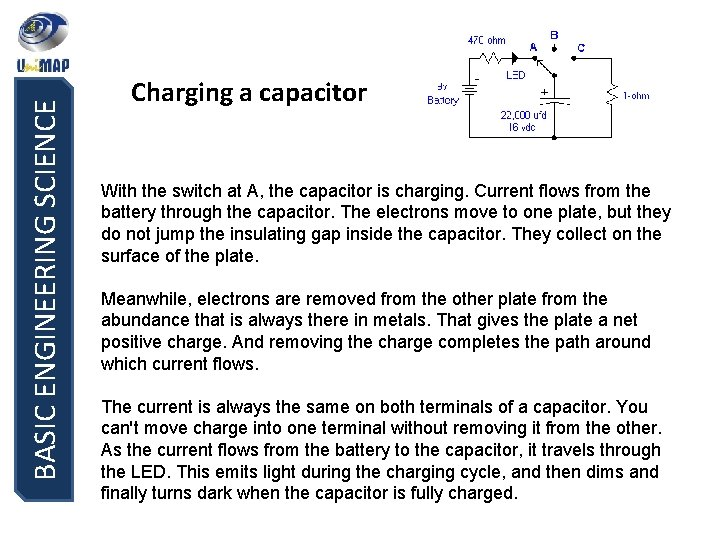BASIC ENGINEERING SCIENCE Charging a capacitor With the switch at A, the capacitor is charging. Current flows from the battery through the capacitor. The electrons move to one plate, but they do not jump the insulating gap inside the capacitor. They collect on the surface of the plate. Meanwhile, electrons are removed from the other plate from the abundance that is always there in metals. That gives the plate a net positive charge. And removing the charge completes the path around which current flows. The current is always the same on both terminals of a capacitor. You can't move charge into one terminal without removing it from the other. As the current flows from the battery to the capacitor, it travels through the LED. This emits light during the charging cycle, and then dims and finally turns dark when the capacitor is fully charged.BASIC ENGINEERING SCIENCE Disconnected With the switch at B, the capacitor is disconnected. What happens? There is no current on one terminal of the capacitor. There must be no current on the other terminal. With no current flowing, the capacitor will keep its 9 -volt charge nearly forever. It is stored in the electric field between the two places. It cannot move due to the insulator -- the charges cannot jump the gap. In practice, no insulator is perfect and the charge will eventually leak away. But this may take months in a high-quality capacitor. Additionally, a significant charge can remain forever, stored in the chemical reaction that ionizes the plate surfaces of an electrolytic capacitorBASIC ENGINEERING SCIENCE Discharging a capacitor With the switch at C, the capacitor is connected to the 1 -ohm resistor. What happens? The charges stored in the capacitor's electric field now have an escape route. They can finally flow from one plate to the other, by travelling through the resistor. The rate of charge travel (current) depends on the circuit resistance, and also on how hard it is pushed by the strength of the internal electric field (voltage).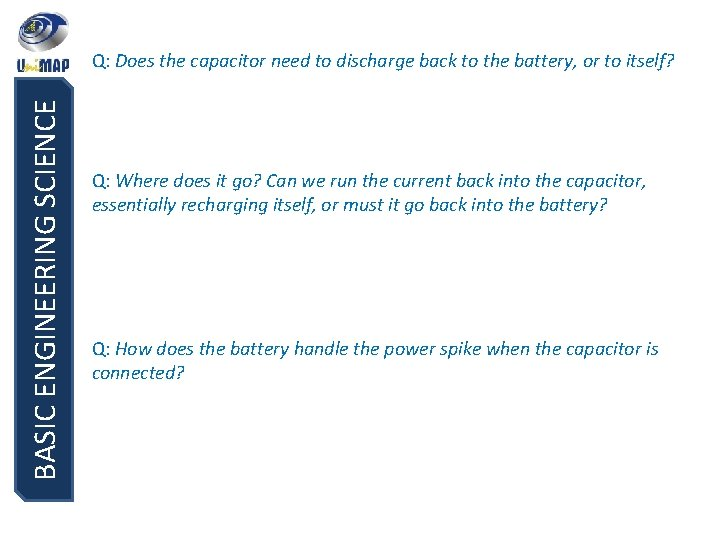BASIC ENGINEERING SCIENCE Q: Does the capacitor need to discharge back to the battery, or to itself? Q: Where does it go? Can we run the current back into the capacitor, essentially recharging itself, or must it go back into the battery? Q: How does the battery handle the power spike when the capacitor is connected?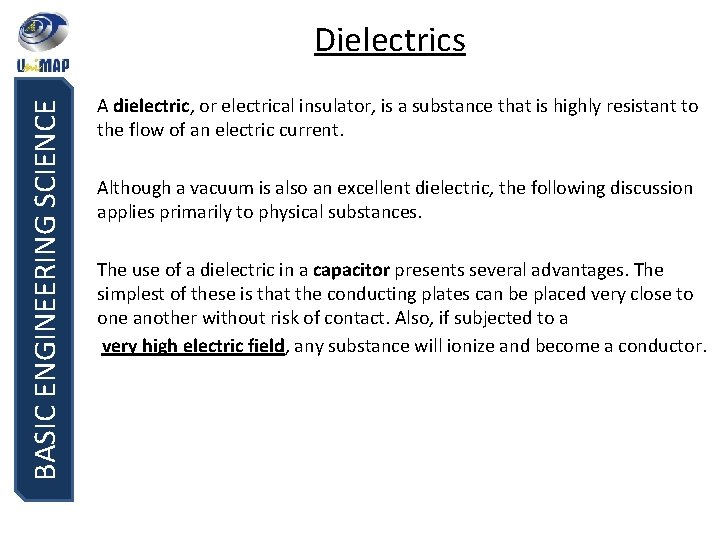BASIC ENGINEERING SCIENCE Dielectrics A dielectric, or electrical insulator, is a substance that is highly resistant to the flow of an electric current. Although a vacuum is also an excellent dielectric, the following discussion applies primarily to physical substances. The use of a dielectric in a capacitor presents several advantages. The simplest of these is that the conducting plates can be placed very close to one another without risk of contact. Also, if subjected to a very high electric field, any substance will ionize and become a conductor.BASIC ENGINEERING SCIENCE Dielectrics “Dielectric” is another word for insulator. A dielectric inside a capacitor increases the capacitor’s energy storage by an amount characterized by the dielectric constant, .BASIC ENGINEERING SCIENCE Dielectrics A dielectric in an electric field becomes polarized; this allows it to sustain a larger electric field for the same potential difference. The net effect: E and V <<, Stored charge remains the same - Capacitance increase.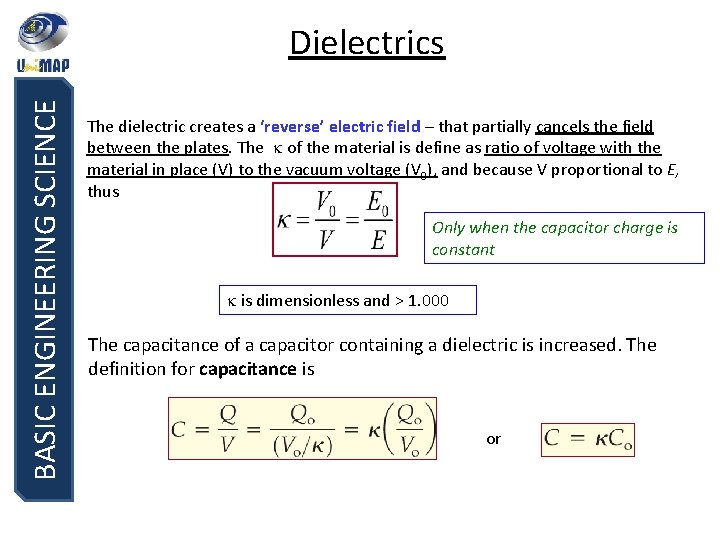BASIC ENGINEERING SCIENCE Dielectrics The dielectric creates a ‘reverse’ electric field – that partially cancels the field between the plates. The of the material is define as ratio of voltage with the material in place (V) to the vacuum voltage (V 0), and because V proportional to E, thus Only when the capacitor charge is constant is dimensionless and > 1. 000 The capacitance of a capacitor containing a dielectric is increased. The definition for capacitance is orBASIC ENGINEERING SCIENCE Dielectrics Inserting a dielectric into a capacitor while either the voltage or the charge is held constant has the same effect – the ratio of charge to voltage increases. Voltage drops Stored energy decrease Charge on a plates increase More energy stored in capacitorBASIC ENGINEERING SCIENCE END OF CHAPTER 6